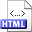Characterizing multiterminal flow networks and computing flows in networks of small treewidth Authors: Torben Hagerup, Jyrki Katajainen, Naomi Nishimura, and Prabhakar Ragde Published in: Journal of Computer and System Sciences 57,3 (1998), 366-375 DOI: 10.1006/jcss.1998.1592 Copyright: © Academic Press Abstract: We show that if a flow network has k input/output terminals (for the traditional maximum-flow problem, k = 2), its external flow pattern (the possible values of flow into and out of the terminals) has two characterizations of size independent of the total number of vertices: a set of 2k + 1 inequalities in k variables representing flow values at the terminals, and a mimicking network with at most 22k vertices and the same external flow pattern as the original network. For the case in which the underlying graph has bounded treewidth, we present sequential and parallel algorithms that can compute these characterizations as well as a flow consistent with any desired feasible external flow (including a maximum flow between two given terminals). For constant k, the sequential algorithm runs in O(n) time on n-vertex networks, and the parallel algorithm runs in O(log n) time on an EREW PRAM with O(n / log n) processors if an explicit tree decomposition of the network of size O(n) is given; if not, known algorithms can compute such a tree decomposition in O((log n)2) time using O(n / (log n)2) processors. Related:HTML (Conference paper) BibLATEX: ```@article{HKNR1998J, author = {Torben Hagerup and Jyrki Katajainen and Naomi Nishimura and Prabhakar Ragde}, title = {Characterizing multiterminal flow networks and computing flows in networks of small treewidth}, journaltitle = {Journal of Computer and System Sciences}, volume = {57}, number = {3}, year = {1998}, pages = {366--375}, } ```